# Betti number

(diff) ← Older revision | Latest revision (diff) | Newer revision → (diff)-dimensional Betti number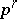of a complexThe rank of the-dimensional Betti group with integral coefficients. For eachthe Betti numberis a topological invariant of the polyhedron which realizes the complex, and it indicates the number of pairwise non-homological (over the rational numbers) cycles in it. For instance, for the sphere: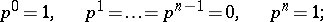for the projective plane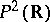: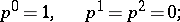for the torus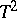: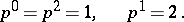For an-dimensional complex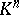the sum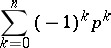is equal to its Euler characteristic. Betti numbers were introduced by E. Betti .

How to Cite This Entry:
Betti number. Encyclopedia of Mathematics. URL: http://encyclopediaofmath.org/index.php?title=Betti_number&oldid=16078
This article was adapted from an original article by M.I. Voitsekhovskii (originator), which appeared in Encyclopedia of Mathematics - ISBN 1402006098. See original article